# Use the Henderson-Hasselbalch equation to calculate the pHpH of each solution: Part A a solution that...

 Use the Henderson-Hasselbalch equation to calculate the pHpH of each solution: Part A a solution that is 0.19 MM in HCHO2HCHO2 and 0.10 MM in NaCHO2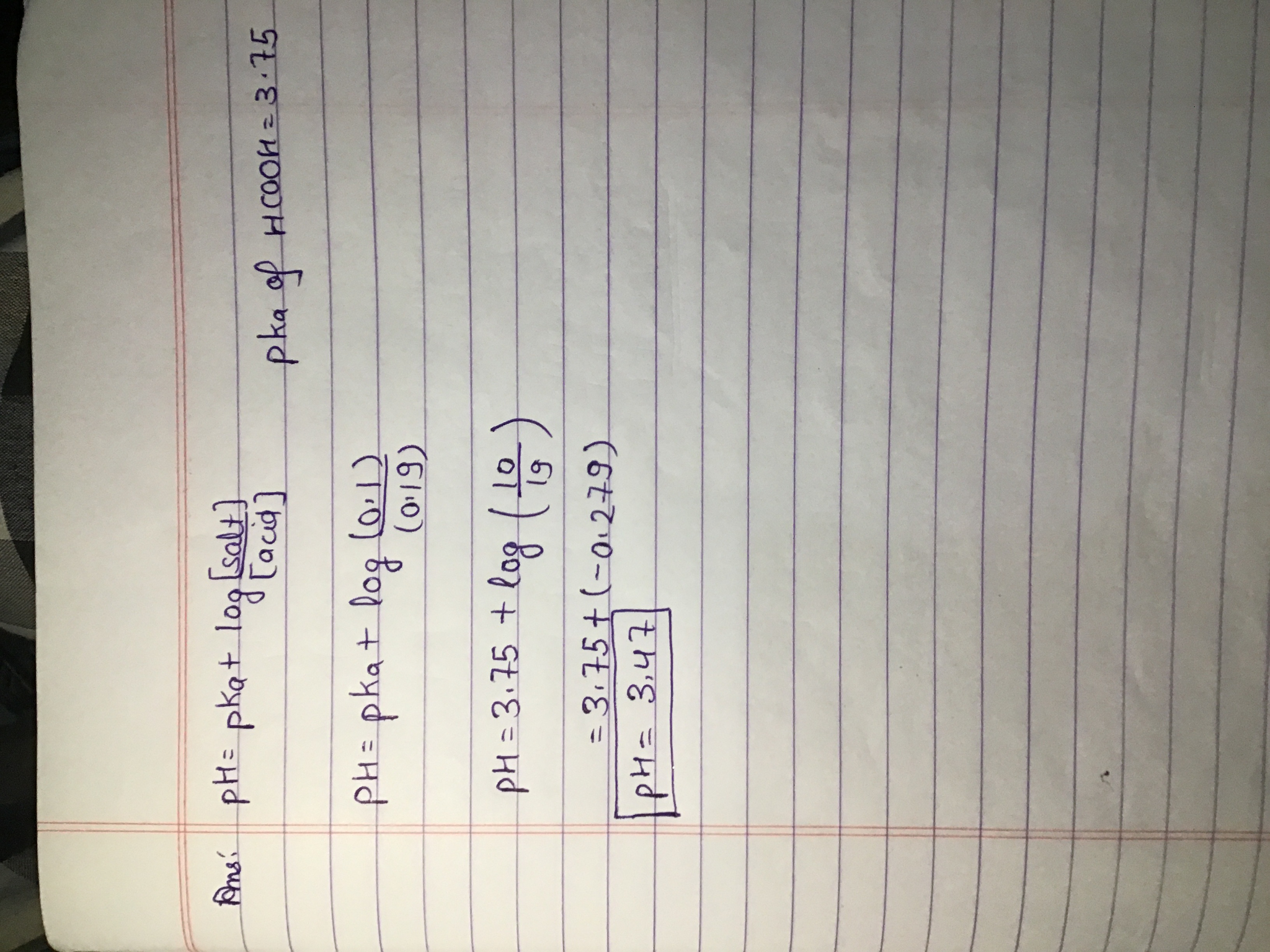#### Earn Coin

Coins can be redeemed for fabulous gifts.

Similar Homework Help Questions
• ### Use the Henderson-Hasselbalch equation to calculate the pHpH of each solution: A) a solution that is...

Use the Henderson-Hasselbalch equation to calculate the pHpH of each solution: A) a solution that is 0.175 MM in HC2H3O2HC2H3O2 and 0.125 MM in KC2H3O2 B) a solution that is 0.190 MM in CH3NH2CH3NH2 and 0.135 MM in CH3NH3Br

• ### Use the Henderson-Hasselbalch equation to calculate the pH of each solution: (a) a solution that is...

Use the Henderson-Hasselbalch equation to calculate the pH of each solution: (a) a solution that is 0.20 M in HCHO2 and 0.10 M in NaCHO2. (Ka=1.8×10−4) (b) a solution that is 0.14 M in NH3 and 0.19 M in NH4Cl. (Kb=1.76×10−5)

• ### Use the Henderson-Hasselbalch equation to calculate the pH of each solution: Part A a solution that...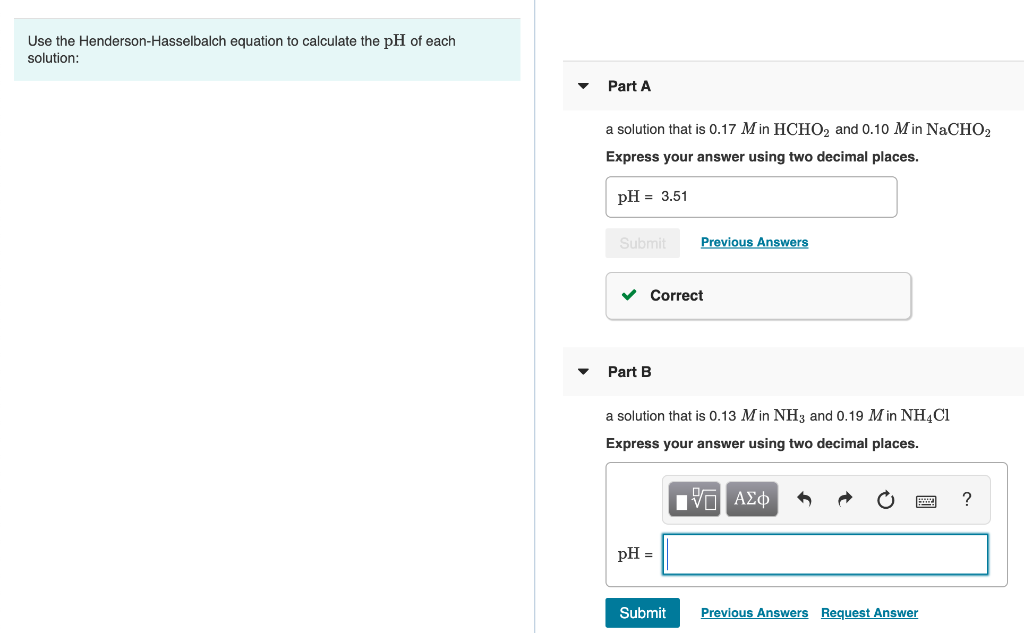Use the Henderson-Hasselbalch equation to calculate the pH of each solution: Part A a solution that is 0.17 Min HCHO2 and 0.10 Min NaCHO2 Express your answer using two decimal places. pH = 3.51 Previous Answers ✓ Correct Part B a solution that is 0.13 Min NH3 and 0.19 M in NH4Cl Express your answer using two decimal places. I AM A O 2 ? pH = | Submit Previous Answers Request Answer

• ### Use the Henderson-Hasselbalch equation to calculate the pH of each solution: A. a solution that is...

Use the Henderson-Hasselbalch equation to calculate the pH of each solution: A. a solution that is 0.14 M in HCHO2 and 0.13 M in NaCHO2 B. a solution that is 0.15 MM in NH3NH3 and 0.18 MM in NH4Cl

• ### Use the Henderson-Hasselbalch equation to calculate the pH of each solution: A. a solution that is...

Use the Henderson-Hasselbalch equation to calculate the pH of each solution: A. a solution that is 0.14 M in HCHO2 and 0.13 M in NaCHO2 B. a solution that is 0.15 M in NH3 and 0.18 M in NH4Cl

• ### calculate pH using Henderson-Hasselbalch equation

Use the Henderson-Hasselbalch equation to calculate the pH of each of the following solutions.a. a solution that is 0.17 in and 0.11 in b. a solution that is 0.13 in and 0.19 in

• ### Use the Henderson-Hasselbalch equation to calculate the pH of each solution: A solution that is 0.14...

Use the Henderson-Hasselbalch equation to calculate the pH of each solution: A solution that is 0.14 M in NH3 and 0.20 M in NH4Cl. (Kb= 1.76x10^-5)

• ### 10. (4 pts. Use the Henderson-Hasselbalch equation to calculate the pH of each solution. d. A...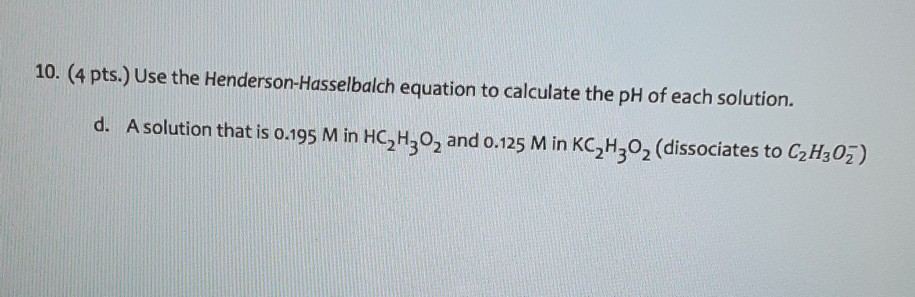10. (4 pts. Use the Henderson-Hasselbalch equation to calculate the pH of each solution. d. A solution that is 0.195 M in HC,H,, and 0.125 Min KC H202 (dissociates to C2H302)

• ### 5. (2pts) To calculate the pH of buffer solution we need to use Henderson-Hasselbalch equation The...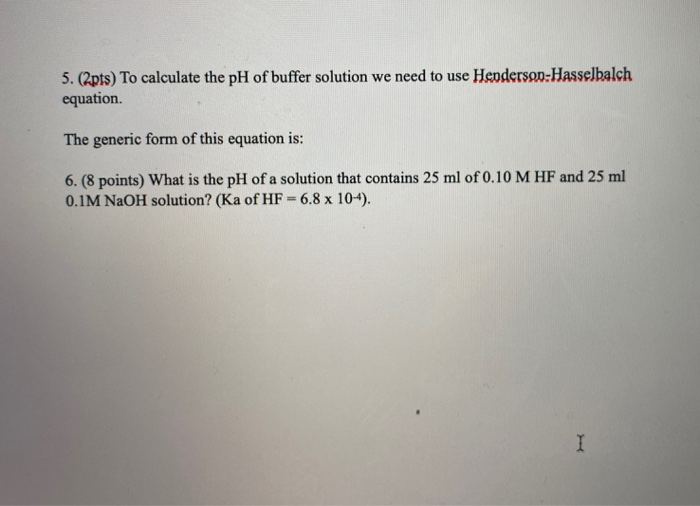5. (2pts) To calculate the pH of buffer solution we need to use Henderson-Hasselbalch equation The generic form of this equation is: 6. (8 points) What is the pH of a solution that contains 25 ml of 0.10 M HF and 25 ml 0.1M NaOH solution? (Ka of HF -6.8 x 104).

• ### Use the Henderson-Hasselbalch equation to calculate the pH of each solution: a solution that contains 0.695%...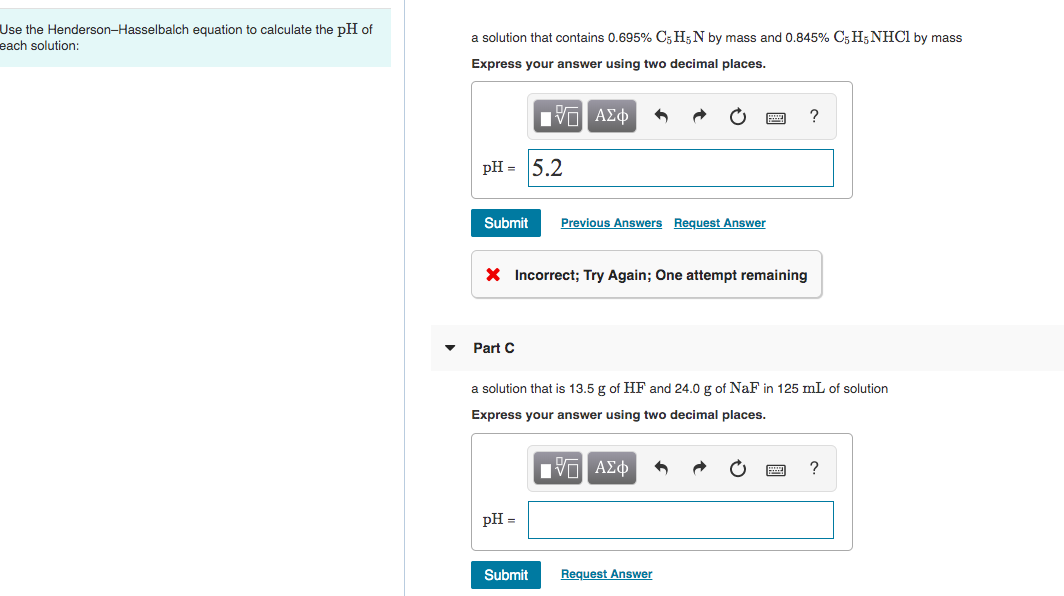Use the Henderson-Hasselbalch equation to calculate the pH of each solution: a solution that contains 0.695% C5H5N by mass and 0.845% C5H5NHCI by mass Express your answer using two decimal places. Hν ΑΣφ ? рH- 15.2 Previous Answers Request Answer Submit Incorrect; Try Again; One attempt remaining Part C a solution that is 13.5 g of HF and 24.0 g of NaF in 125 mL of solution Express your answer using two decimal places. ΑΣφ ? pH Request Answer Submit